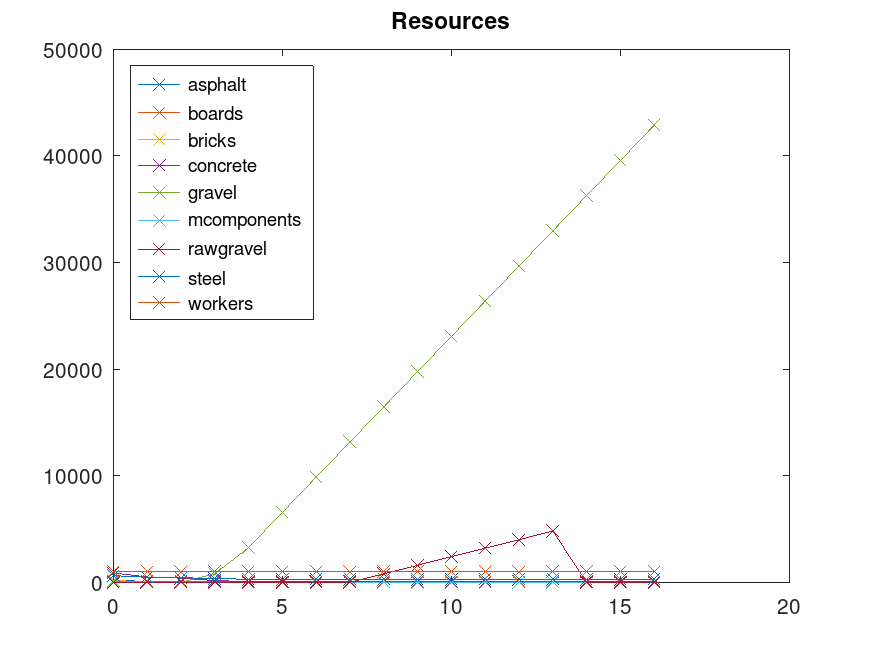# Workers and Resources parser published

## Overview

What the program does is parse the building definition files for all the buildings in the game to figure out two things:

• how much resources and labour it takes to build them
• what the buildings consume and produce, also known as their technical coefficients

I haven't updated the code since 2021, but since the game is nearing its official release perhaps I will take another look at it.

## Data formats

Let's take a closer look at the data files related to buildings, which are located in media_soviet/buildings_types. Let's look at the gravel processing plant, which consists of three files:

• gravel_processing.ini
• gravel_processing.bbox
• gravel_processing.fire

Let's ignore gravel_processing.fire because my code doesn't use it.

### .bbox files

gravel_processing.bbox defines multiple bounding boxes which have names like techShape5 and concreteShape1. The files are little-endian and the first four bytes are a 32-bit integer specifying the number of bounding boxes in the file. Each bounding box is exactly 540 bytes and consists of the following:

• name (512 bytes, string)
• index (4 bytes, uint32, counts up from zero)
• xmin, ymin, zmin, xmax, ymax, zmax (4*6 = 24 bytes, float32)

The names are NUL terminated but often not NUL filled, so there may be interesting strings in them. The index doesn't appear to be useful. The six floating point values define the bounding box; minimum and maximum in all three cardinal directions.

### .ini files

The .ini files are text and look like this:

$NAME 6158$VEHICLE_STATION 65.4619 0 -15.1333 65.4619 0 3.5876
$VEHICLE_STATION 68.4619 0 -15.1333 68.4619 0 3.5876$TYPE_FACTORY

$WORKERS_NEEDED 15$PRODUCTION gravel 5.5
$CONSUMPTION rawgravel 8.0$CONSUMPTION_PER_SECOND eletric 0.4
[snip]


Just from the strings we can get the gist of what the file is doing. This file specifies a factory that consumes raw gravel and electricity and outputs gravel. The rates of consumptions are also given, and are per worker multiplied by some factor to give a per second rate. There is also a spelling error in "eletric" (should be "electric") which has thrown me off many times.

For most buildings the cost to build them aren't actually given explicitly. Instead they are derived from the bounding boxes mentioned earlier. For example the gravel processing plant has these lines (heavily abbreviated):

$COST_WORK SOVIET_CONSTRUCTION_GROUNDWORKS 0.0$COST_RESOURCE_AUTO ground_asphalt      1.0
$COST_WORK SOVIET_CONSTRUCTION_SKELETON_CASTING 1.0$COST_RESOURCE_AUTO wall_concrete 0.8
$COST_WORK SOVIET_CONSTRUCTION_STEEL_LAYING 1.0$COST_RESOURCE_AUTO wall_steel 0.35


What these do is tell the game to compute the resources required to build the plant from a bunch of formulas that operate on relevant bounding boxes. I had initially intended to reverse engineer these formulas using least squares fitting, but I reached out to 3DIVISION first and got a reply from Peter Adamcik who gratiously provided me with a snippet of the actual C++ code that computes these automatic costs. Thanks Peter!

The ground area, the wall area and the volume of each relevant bounding box are summed up into three separate variables that I have dubbed $g$, $w$ and $v$ respectively. The first argument in \$COST_RESOURCE_AUTO then says which formula to use. For example ground_asphalt, which is the foundation of the plant, uses $k=g/300+0.08v/3000$ and $150k$ workdays, $13k$ tons of concrete, $10k$ tons of gravel and $8k$ tons of asphalt. The second argument (1.0) is a further scaling factor. This step (called SOVIET_CONSTRUCTION_GROUNDWORKS) has to be completed before the construction crew can start on the next phase of construction (SOVIET_CONSTRUCTION_SKELETON_CASTING).

Some buildings need extra resources that are given explicitly in the .ini file.

## lp_solve generator

Another part of the code (generate_lp.py) generates linear programs in lp_solve format. These are fed into lp_solve and the resulting solution can then be further processed. It is in the generator that the main experimentation happens. One can set up scenarios like "maximize gravel production over 1 year" or "maximize dollar revenue from exports over 10 years". Time is taken into account, as is the fact that one has to build a whole plant before it can be put to use. The latter is an integer constraint and makes the programs mixed integer programs.

## Results parser and GNU Octave code generator

parse_result.py performs two tasks: parsing the output of lp_solve and generating GNU Octave code to plot it. Yes I like writing generators.

## run.sh

run.sh runs the whole shebang and outputs plots like this one:The goal in this case was to maximize gravel by the last time step. Production of raw gravel is stopped at time step 14 so as to reassign workers to gravel processing.